# How to calculate gasfluxes

#### 2018-08-04

This vignette introduces the gasfluxes package to calculate soil greenhouse gas fluxes from static chamber measurements. The package offers different models for measured concentration-time relationships from static chambers within the function gasfluxes

• “linear”
• “robust linear”
• “HMR” (see Pedersen et al., 2010)
• “original HMR” (the implementation according to Pedersen et al. 2010 in the HMR package)
• “NDFE”

and offers the function selectfluxes to use selection algorithms that combine the different models appropriately

## Data import

The input data.frame or data.table can be imported easily from a CSV file (e.g., as exported by Excel):

library(data.table)
#adjust path (see help("setwd")) and file name as appropriate
#here we use two flux measurements from the file as an example
fluxMeas <- fluxMeas[ID %in% c("ID6","ID11")]

fluxMeas
#>      ID        V A      time         C
#> 1:  ID6 0.535125 1 0.0000000 0.3241982
#> 2:  ID6 0.535125 1 0.3333333 0.3838311
#> 3:  ID6 0.535125 1 0.6666667 0.3623941
#> 4:  ID6 0.535125 1 1.0000000 0.5067303
#> 5: ID11 0.550000 1 0.0000000 0.3337777
#> 6: ID11 0.550000 1 0.3333333 0.4410218
#> 7: ID11 0.550000 1 0.6666667 0.5080499
#> 8: ID11 0.550000 1 1.0000000 0.5417371

The ID column must be a unique identifier for each flux measurement, hence the same value for all the concentration-time points that belong to the same flux. Column V stands for the chamber volume, A for the chamber area, time for the elapsed time after chamber closure and C is the concentration of the measured species (CO2, N2O, CH4 etc.)

Units of the output fluxes depend on input units. It’s recommended to use [V] = m3, [A] = m2, [time] = h, [C] = [mass or mol]/m3, which results in [f0] = [mass or mol]/m2/h. Since all algorithms only use V/A, A can be input as 1 and V as the chamber height.

The following features of the input will be checked for sanity:

• if specified columns exist (ID,V,A,time,C)
• if input is numeric
• if there are any NA’s
• non-unique values in V or A per chamber and if time is sorted and time values are unique
• only positive input is allowed

## Calculating fluxes with the gasfluxes function

If the input dataframe has the appropriate format, plug it into the function to calculate the fluxes:

library(gasfluxes)
flux.results <- gasfluxes(fluxMeas, method = c("linear","robust linear", "HMR"), plot = TRUE)
#> ID6: lm fit successful
#> ID6: rlm fit successful
#> ID6: HMR fit failed
#> ID11: lm fit successful
#> ID11: rlm fit successful
#> ID11: HMR fit successful
flux.results
#>      ID linear.f0 linear.f0.se linear.f0.p linear.C0 linear.AIC linear.AICc
#> 1:  ID6 0.0844683   0.03531910  0.13923269 0.3153645  -9.516839         Inf
#> 2: ID11 0.1139995   0.01920685  0.02723196 0.3525107 -14.609437         Inf
#>    linear.RSE  linear.r linear.diagnostics robust.linear.f0 robust.linear.f0.se
#> 1: 0.04919468 0.8607673                          0.09719292         0.002805431
#> 2: 0.02602898 0.9727680                          0.11399952         0.019206850
#>    robust.linear.f0.p robust.linear.C0 robust.linear.weights
#> 1:       0.0006741157        0.3232908           1|1|0.033|1
#> 2:       0.0272319550        0.3525107               1|1|1|1
#>    robust.linear.diagnostics    HMR.f0  HMR.f0.se   HMR.f0.p HMR.kappa  HMR.phi
#> 1:                                  NA         NA         NA        NA       NA
#> 2:                           0.2332107 0.01194175 0.03257022  1.628399 0.593832
#>      HMR.AIC HMR.AICc     HMR.RSE
#> 1:        NA       NA          NA
#> 2: -33.15832       NA 0.002821234
#>                                                    HMR.diagnostics
#> 1: Missing value or an infinity produced when evaluating the model
#> 2:

The output of gasfluxes contains the estimate of the initial flux (f0) for each method chosen (linear, robust linear and HMR in this case) including statistical parameters of the fits:

• the standard error se
• p-value
• estimated concentration at time zero C0
• Akaike Information Citerion
• Akaike Information Citerion corrected for finite sample size AICc (needs more than four points per flux)
• the residual standard error RSE
• for linear regression the R value is shown (not squared!)
• Diagnostic information about the fit
• estimated parameters depending on the fit (note HMR.kappa will be used in the decision scheme kappa.max)

For the improved HMR fitting function the starting value k_HMR = log(1.5) is used by default. This is appropriate for hourly values. If you change the input i.e. by providing your measurement time in seconds use k_HMR = log(1.5/3600).

It is possible to have more than one ID column in the input file. For instance, it is often convenient to use a plot_ID and a date column by specifying the column names, e.g., gasfluxes(fluxMeas, .id = c("plot_ID", "date"), method = c("linear","robust linear", "HMR")).

###Graphical output

By default and with plot = TRUE the flux calcuation function plots a figure for each chamber flux that is stored in the subfolder /pics in the working directory. The following example on the left shows a flux where HMR could not be fitted and the 3rd concentration is regarded as outlier by the robust linear estimate. The second examples shows a flux that is well fitted by HMR which increases the flux estimate twofold compared to the linear fit (see numbers in the output above; HMR.f0/linear.f0).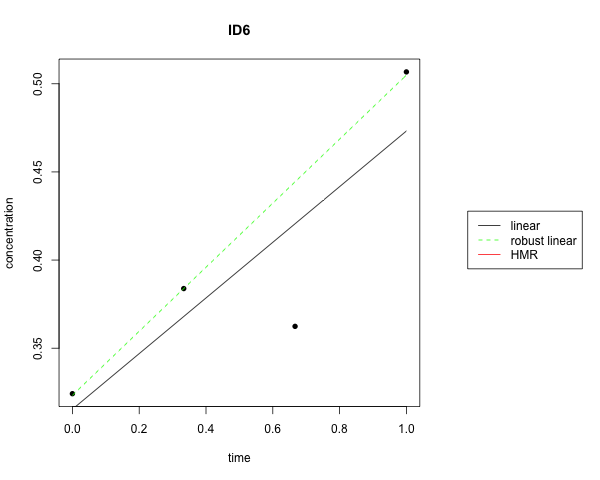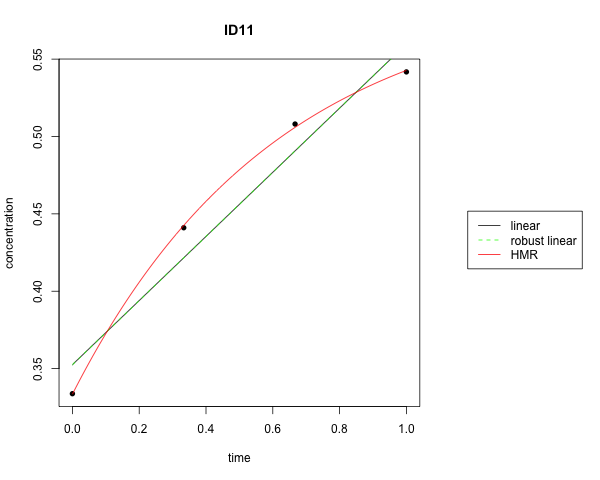## Applying selection algorithm with the selectfluxes function

It is not apriori obvious which of the flux estimates should be selected for an individual chamber measurement. In case of small fluxes and large relativ measurement uncertainty linear estimates are the most appropriate choice. However, if non-linear effects (like decreasing diffusion gradient, lateral gas flow and chamber leakage) impact the increase in concentration within the chamber, the non-inear estimate of the HMR model is a better choice. The selectfluxes function offers some well defined decision algorithms that avoid the need for manual decisions (like suggested in the original HMR package). The most extensively tested decision ruleset is kappa.max, which optimises the balance between bias and uncertainty by taking the minimal detectable flux of the current system (f.detect see its suggested calculation below) and the size of the flux into account (see Hüppi et al. (2018) for details). t.meas is the time of the final concentration time point of the individual chamber measurement. To apply the kappa.max selection to your calculated fluxes use the selectfluxes function the following way:

selectfluxes(flux.results, select = "kappa.max", f.detect = 0.031, t.meas = 1)
#>      ID linear.f0 linear.f0.se linear.f0.p linear.C0 linear.AIC linear.AICc
#> 1:  ID6 0.0844683   0.03531910  0.13923269 0.3153645  -9.516839         Inf
#> 2: ID11 0.1139995   0.01920685  0.02723196 0.3525107 -14.609437         Inf
#>    linear.RSE  linear.r linear.diagnostics robust.linear.f0 robust.linear.f0.se
#> 1: 0.04919468 0.8607673                          0.09719292         0.002805431
#> 2: 0.02602898 0.9727680                          0.11399952         0.019206850
#>    robust.linear.f0.p robust.linear.C0 robust.linear.weights
#> 1:       0.0006741157        0.3232908           1|1|0.033|1
#> 2:       0.0272319550        0.3525107               1|1|1|1
#>    robust.linear.diagnostics    HMR.f0  HMR.f0.se   HMR.f0.p HMR.kappa  HMR.phi
#> 1:                                  NA         NA         NA        NA       NA
#> 2:                           0.2332107 0.01194175 0.03257022  1.628399 0.593832
#>      HMR.AIC HMR.AICc     HMR.RSE
#> 1:        NA       NA          NA
#> 2: -33.15832       NA 0.002821234
#>                                                    HMR.diagnostics kappa.max
#> 1: Missing value or an infinity produced when evaluating the model  2.724784
#> 2:                                                                  3.677404
#>          flux     flux.se       flux.p        method
#> 1: 0.09719292 0.002805431 0.0006741157 robust linear
#> 2: 0.23321066 0.011941753 0.0325702174           HMR
flux.results[,c(1,26:30)]
#>      ID kappa.max       flux     flux.se       flux.p        method
#> 1:  ID6  2.724784 0.09719292 0.002805431 0.0006741157 robust linear
#> 2: ID11  3.677404 0.23321066 0.011941753 0.0325702174           HMR

This appends the columns shown above with the selected and recommanded flux estimate and its method used.

### Estimate the minimal detectable flux f.detect

The minimal detectable flux relevant for kappa.max selection algorithm depends on the measurement precision of the GC (GC.sd), the chamber size (area A and volume V) and the timing of the sampling scheme (t i.e. at 0, 20, 40 and 60 minutes).

### estimate f.detect by simulation
C0    <- 325   #ambient concentration, here in [ppm]
GC.sd <- 5 #uncertainty of GC measurement, here in [ppm]
#create simulated concentrations corresponding to flux measurements with zero fluxes:
set.seed(42)
sim <- data.frame(t = seq(0, 1, length.out = 4), C = rnorm(4e3, mean = C0, sd = GC.sd),
id = rep(1:1e3, each = 4), A = 1, V = 0.535125)   # specify your sampling scheme t (here in [h]) and chamber volume (V) and area (A)
#fit HMR model:
simflux <- gasfluxes(sim, .id = "id", .times = "t", methods = c("HMR", "linear"), plot = FALSE, verbose = F)
simflux[, f0 := HMR.f0]
simflux[is.na(f0), f0 := linear.f0] # use linear estimates where HMR could not be fitted
#dection limit as 97.5 % quantile (95 % confidence):
f.detect <- simflux[, quantile(f0, 0.975)]
f.detect # here in [ppm/h/m^2], use same unit as your flux estimates,
#>    97.5%
#> 28.16894
#e.g., convert to mass flux assuming chamber temperature of 15 degree celcius and standard air pressure
f.detect / 1000 * 28 * 273.15 / 22.4 / (273.15 + 15)
#>      97.5%
#> 0.03337822

## Visualise the flux calculation procedure for a specific GHG chamber measurement system

Simulations are useful for understanding the behaviour of flux calculation algorithms. The performance of a flux calculation scheme depends on the precision of the GC (GC.sd), height of the chamber (V/A), the sampling time scheme (especially the chamber closure time, refered as t.meas), the tightness of the chambers, the reliability of the sampling vial handling etc. This is why it makes sense to look at each specific measurement system and how well it copes with non linear flux estimates (i.e. HMR).

With a simulation we can estimate the impact of the calculation scheme on the flux itself and hence its bias: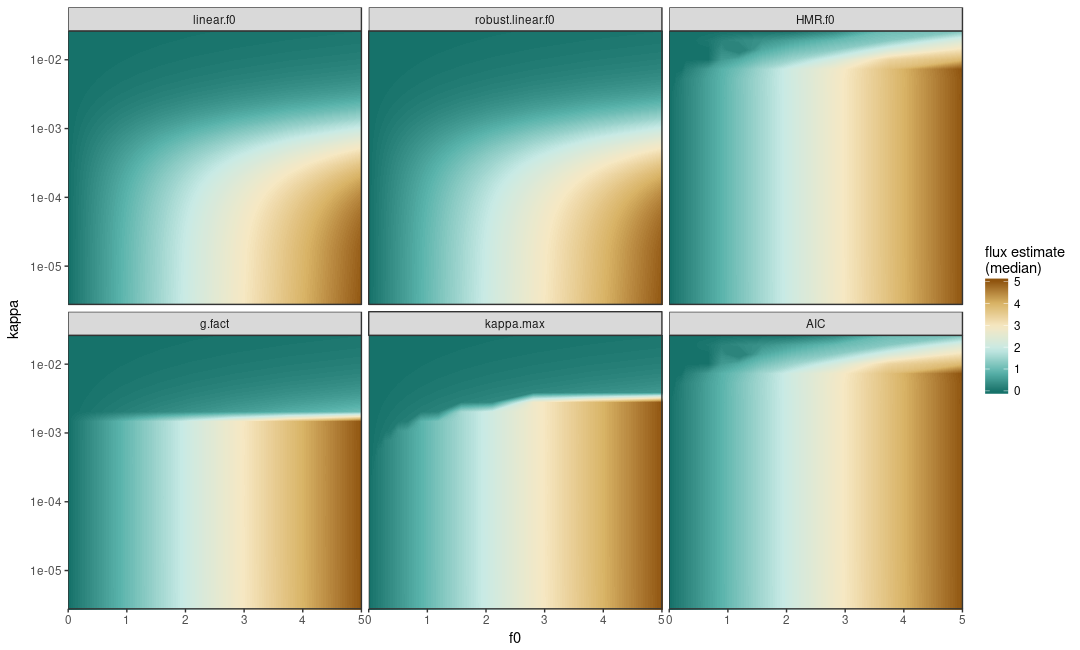and the uncertainty associated with it: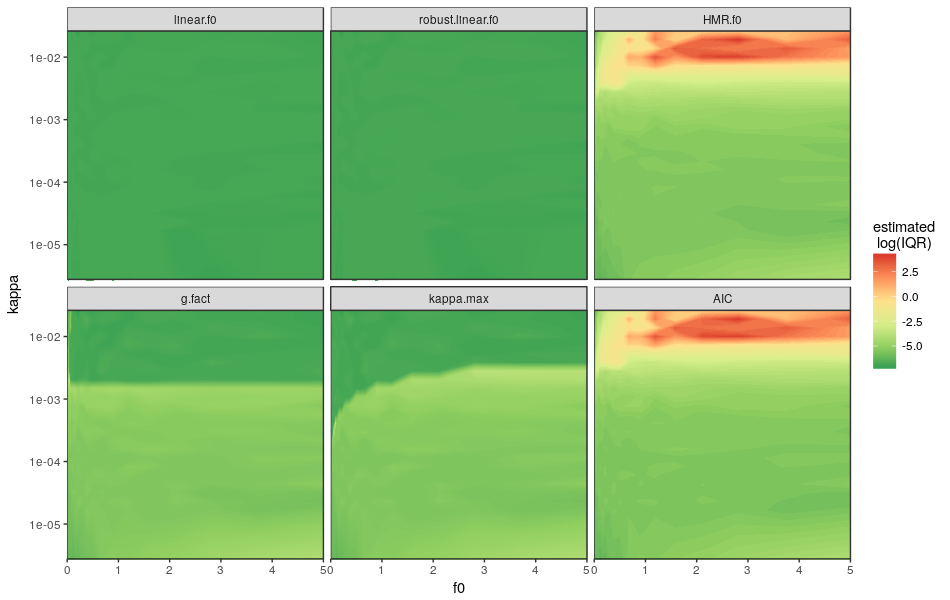Once having created a simulated flux dataset one can check, where the fluxes are placed in the parameter space of the estimated kappa vs. flux f0.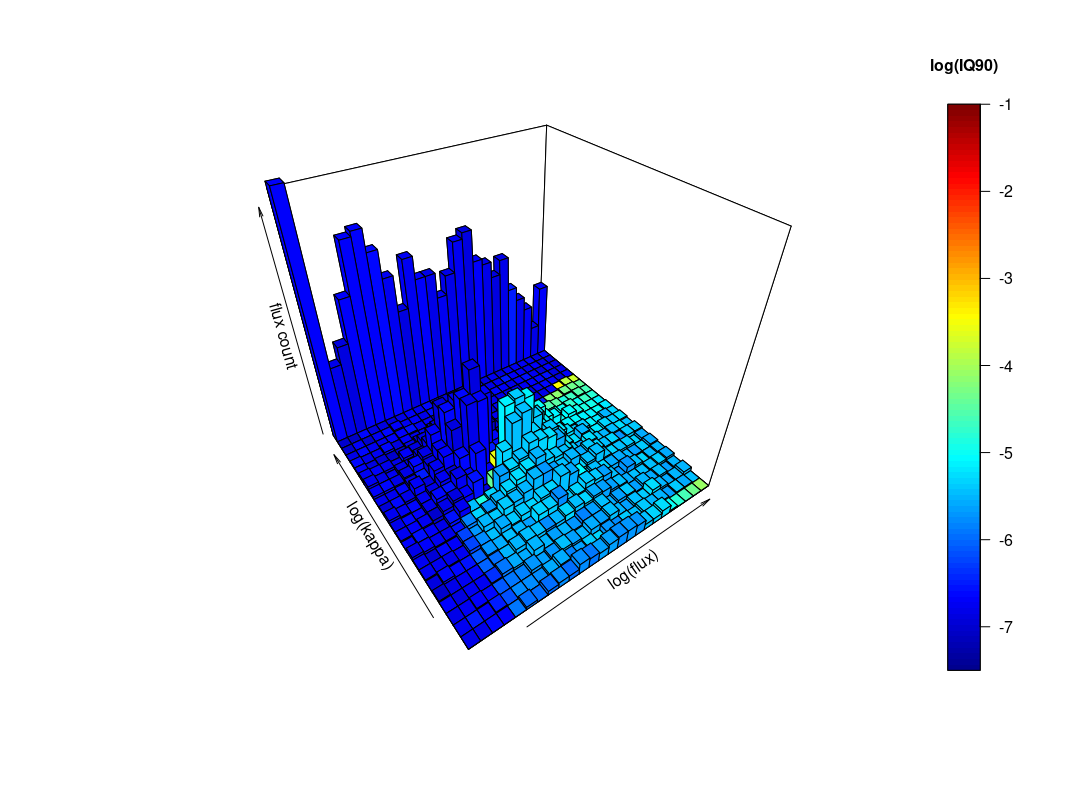The code for the example simulation is available as a Gist and further details are described in Hüppi et al. (2018).